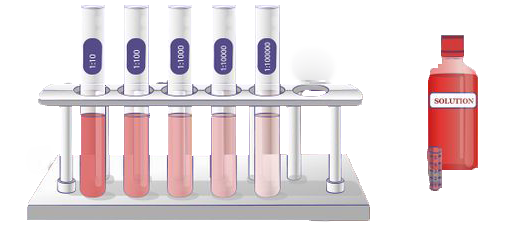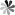## Solution Dilution Calculator

Dilute Solution of Known Molarity

Calculate now!

* Stock concentration:
* Desired final volume:
* Desired concentration:
L* Our calculator is based on the following equation:
Concentration (start) x Volume (start) = Concentration (final) x Volume (final)
It is commonly abbreviated as: C1V1 = C2V2

For example:
What volume of a given 10 mM stock solution is required to make 20ml of a 90 μM solution?
With the equation C1V1 = C2V2, where C1=10 mM, C2=90 μM, V2=20 ml and V1 is the unknown:

1. Enter 10 into the Stock Concentration (start) box and select the correct unit (millimolar)
2. Enter 90 into the Desired Concentration (final) box and select the correct unit (micromolar)
3. Enter 20 into the Desired Volume (final) box and select the correct unit (milliliter)
4. Press calculate
5. The answer of 180 microliter (0.18ml) appears in the Required Volume (start) boxLoading ......